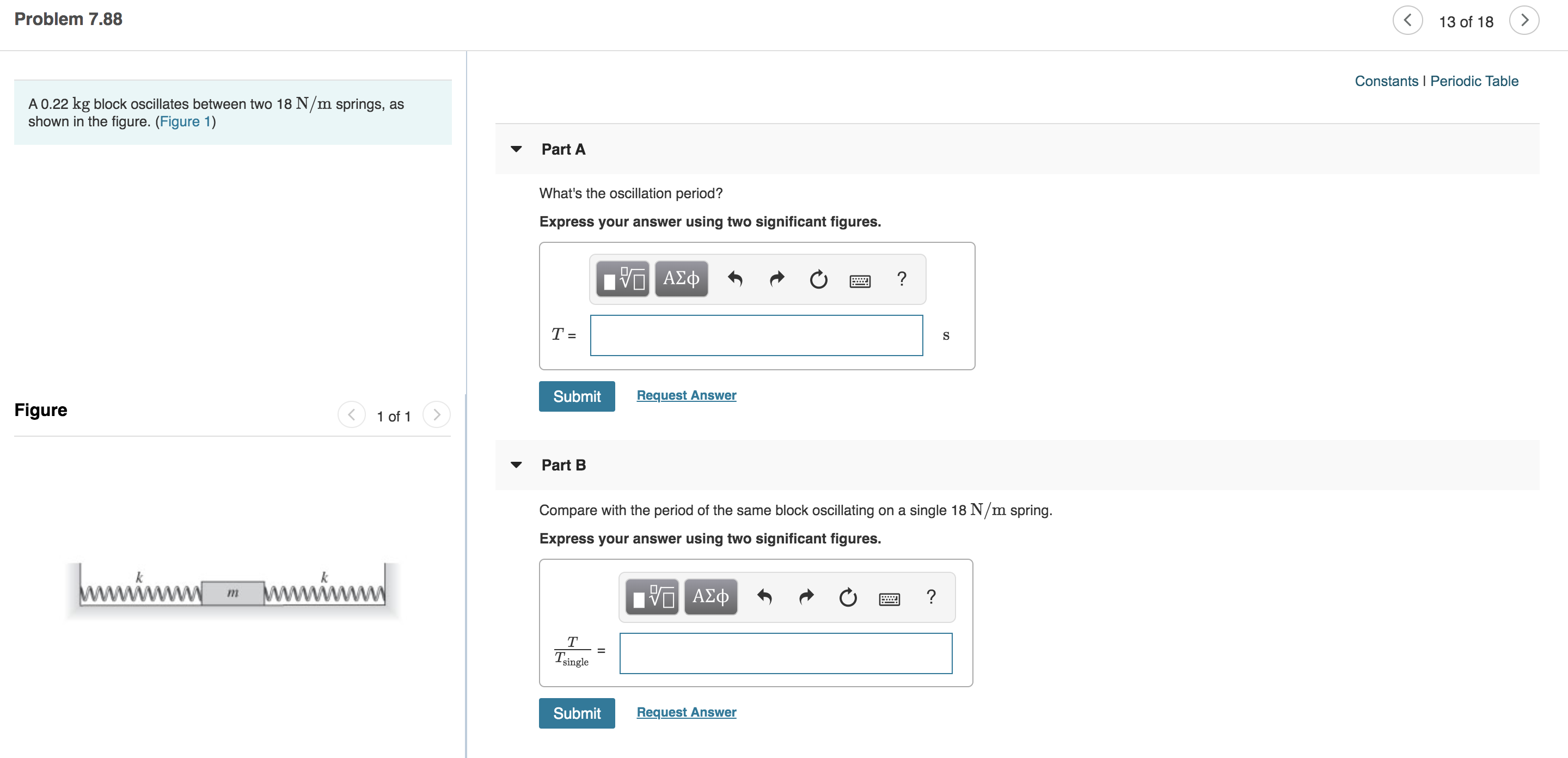# Problem 7.88 < 13 of 18 Constants Periodic Table A 0.22 kg block oscillates between two 18 N/m springs, as shown in the figure. (Figure 1) Part A What's the oscillation period? Express your answer using two significant figures. ν ΑΣφ ? Т- Request Answer Submit Figure 1 of 1 Part B Compare with the period of the same block oscillating on a single 18 N/m spring Express your answer using two significant figures. www.wnv ww ΑΣφ Tsingle Request Answer Submit

Question

Part A,Bhelp_outlineImage TranscriptioncloseProblem 7.88 < 13 of 18 Constants Periodic Table A 0.22 kg block oscillates between two 18 N/m springs, as shown in the figure. (Figure 1) Part A What's the oscillation period? Express your answer using two significant figures. ν ΑΣφ ? Т- Request Answer Submit Figure 1 of 1 Part B Compare with the period of the same block oscillating on a single 18 N/m spring Express your answer using two significant figures. www.wnv ww ΑΣφ Tsingle Request Answer Submit fullscreen

### Want to see this answer and more?

Experts are waiting 24/7 to provide step-by-step solutions in as fast as 30 minutes!*

*Response times vary by subject and question complexity. Median response time is 34 minutes and may be longer for new subjects.
Tagged in
SciencePhysics

### Wave Motion